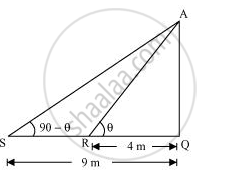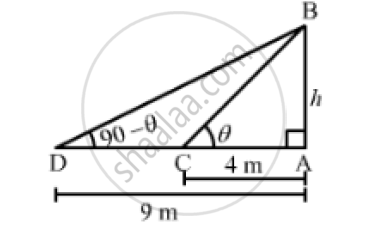# He Angles of Elevation of the Top of a Tower from Two Points at a Distance of 4 M and 9 M. from the Base of Tower and in Same Straight Line with It Are Complementary. Rove that the Height of the Towe - Mathematics

The angles of elevation of the top of a tower from two points at a distance of 4 m and 9 m. from the base of the tower and in the same straight line with it are complementary. Prove that the height of the tower is 6 m.

#### Solution 1Let AQ be the tower and R, S are the points 4m, 9m away from the base of the tower respectively.

The angles are complementary. Therefore, if one angle is θ, the other will be 90 − θ.

In ΔAQR,

AQ/QR = tanΘ

AQ/4 = tanΘ    ... 1

In ΔAQS,

AQ/SQ = tan(90 - Θ)

AQ/9 = cot Θ ...2

On multiplying equations (i) and (ii), we obtain

(AQ/4)(AQ/9) = (tanΘ).(cot Θ)

(AQ^2)/36 = 1

AQ^2 = 36

AQ = sqrt36 = +-6

However, height cannot be negative.

Therefore, the height of the tower is 6 m

#### Solution 2

Let AB be the tower and C and D be two points such that AC = 4m and AD  9m.
Let:
AB = hm, ∠BCA=theta  and ∠BDA=  90° - thetaIn the right ΔBCA,we have:

tan theta = (AB)/(AC)

⇒ tan theta = h/4            ...............(1)

In the right  ΔBDA,we have:

 tan (90° - theta ) = (AB) /(AD)

⇒ cot theta = h/9                     [ tan (90° - theta ) = cot theta]

⇒1/ tan theta = h/9            ................(2)       [ cot theta = 1/ tan theta]

Multiplying equations (1) and (2), we get

tan theta xx 1/ tan theta = h/4xxh/9

⇒ 1=( h^2)/36

⇒ 36=h^2

⇒h = +-6

Height of a tower cannot be negative
∴Height of the tower = 6 m

Concept: Heights and Distances
Is there an error in this question or solution?

#### APPEARS IN

NCERT Class 10 Maths
Chapter 9 Some Applications of Trigonometry
Exercise 9.1 | Q 16 | Page 205
RD Sharma Class 10 Maths
Chapter 12 Trigonometry
Exercise 12.1 | Q 67 | Page 34
RS Aggarwal Secondary School Class 10 Maths
Chapter 14 Height and Distance
Exercises | Q 29

Share